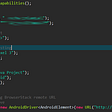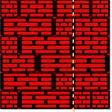# A DP problem solved by using a Knapsack problem way

This is this week’s biweekly contest problem. The solution is pretty similar to the Knapsack problem.

# Explanation

`words = ["acca","bbbb","caca"], target = "aba"`

we firstly can get the following information

for the character ‘a’, for the location of different indexes, it has the following number of words:

for a:

index 0: [‘acca’]

index 1: [‘caca’]

index 2: []

index 3: [‘acca’, ‘caca’]

for b:

index 0: [‘bbbb’]

index 1: [‘bbbb’]

index 2: [‘bbbb’]

index 3: [‘bbbb’]

For the above example, we can have a DP table as below

`    0     1      2       3 a.  1     1      0       2  b.  0     1*1.   1*(1+1) 1*(1+1+0)a   0     0.     0*(...) 2*(0+1+2)`

We can use a natively 2D DP without optimization.

# A naively 2D DP without optimization

It has the time complexity of O(mn*m) as we don’t have the optimization.

It got TLE.

85 / 89 test cases passed.

`# time complexity O(mnm), space O(mn)class Solution:    def numWays(self, words: List[str], target: str) -> int:        s = set(target)        d = collections.defaultdict(lambda : collections.defaultdict(int))        for word in words:            for i, char in enumerate(word):                if char in s:d[char][i] += 1        n = len(target)        m = len(words)                dp = [*m for _ in range(n)]        dp = [d[target][j] for j in range(m)]        for i in range(1, n):            for j in range(i, m):                dp[i][j] = d[target[i]][j]*sum(dp[i-1][k] for k in range(j))        return sum(dp[-1])%(10**9 + 7)`

# A naively 2D DP with time complexity optimization

We can use a prefix sum to optimize the time complexity as O(mn)

`# time complexity O(mn), space O(mn)class Solution:    def numWays(self, words: List[str], target: str) -> int:        s = set(target)        d = collections.defaultdict(lambda : collections.defaultdict(int))        for word in words:            for i, char in enumerate(word):                if char in s:d[char][i] += 1        n = len(target)        m = len(words)                dp = [*m for _ in range(n)]        dp = [d[target][j] for j in range(m)]        for k in range(1, m):            dp[k] += dp[k-1]        for i in range(1, n):            for j in range(i, m):                dp[i][j] = d[target[i]][j]*dp[i-1][j-1]            for k in range(i + 1, m):                dp[i][k] += dp[i][k-1]        return dp[-1][-1]%(10**9 + 7)`

# Bottom UP DP with time/space complexity optimization

`# time complexity O(mn), space O(m)class Solution:    def numWays(self, words: List[str], target: str) -> int:        s = set(target)        d = collections.defaultdict(lambda : collections.defaultdict(int))        for word in words:            for i, char in enumerate(word):                if char in s:d[char][i] += 1        n = len(target)        m = len(words)                dp = *m        for i in range(n):            new_dp = *m            for j in range(i, m):                new_dp[j] = dp[j-1]*d[target[i]][j]            for k in range(i, m):                if k > 0: new_dp[k] += new_dp[k-1]            dp = new_dp        return dp[-1]%(10**9 + 7)`

--

--

--

## More from Jimmy Shen

Data Scientist/MLE/SWE @takemobi

Love podcasts or audiobooks? Learn on the go with our new app.

## Deploying the Stylegan2 Project using Nivida RTX 3080 and TensorFlow 1.x## Running Automation Test in Device Farm## Integrate Twilio, Stripe API and Laravel## PUBLIC LAUNCH IS NOW BEING ON AIR!## Time to Update the Agile Principles## How Microsoft Visual Studio 2017 once had a feature of silent deleting of the user files## Jimmy Shen

Data Scientist/MLE/SWE @takemobi

## Leetcode Q554. Brick Wall (Q463)## Leetcode Solutions — 2Sums